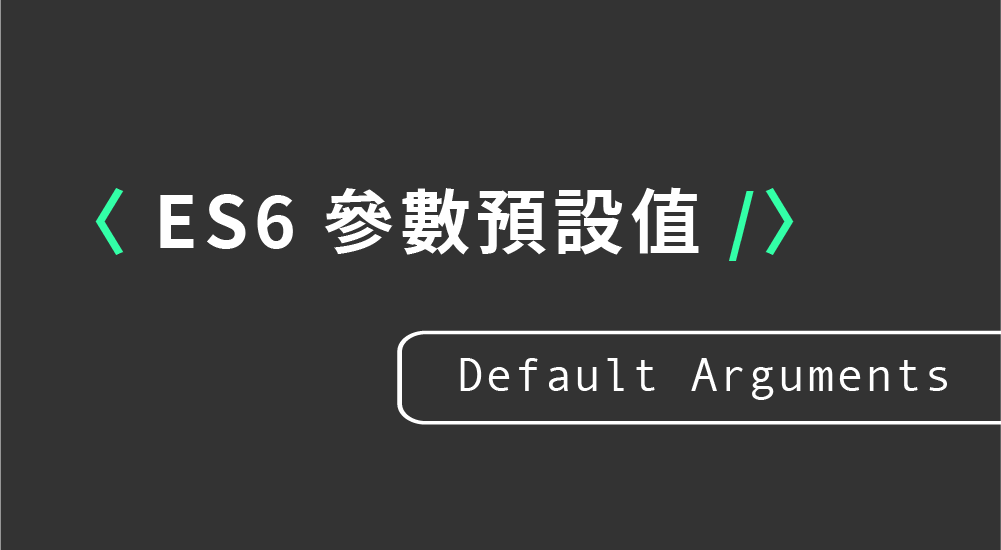#DAY 6
1
Modern Web

## Day06【ES6 小筆記】函式參數預設值 - 基礎範例（Default Arguments）### 參數預設值

ES6 提供了更簡易的函式參數預設值的設定方式，讓我們可以更直覺的來進行設定，優點如下：

• 減少程式碼
• 有利於代碼的可讀性

#### 在過去，設定函式預設的參數值時，需要這樣寫：

``````function count(a,b) {
a = a || 2;
b = b || 3;
return a + b;
};

console.log(count(6,3)); // 9
console.log(count()); // 5 因為沒有代入參數，所以會出現預設的a=2,b=3
``````

#### 而在 ES6 之後，提供了一個更簡單的方法：

``````function count(x = 3, y = 2){
console.log(x+y);
}
count(); // 5
``````
##### - 如果省略帶入參數的話，丟入的參數值會`由前往後`代入
``````//只帶入一個參數
function count(x = 3, y = 2){
console.log(x+y);
}
count(5); // 7

//只有第一個參數有預設值
function count(x = 3, y){
console.log(x+y);
}
count(5); // Nan
count(2,1); // 3

//只有第二個參數有預設值
function count(x, y=3){
console.log(x+y);
}
count(2); // 5
count(2,6); // 8
``````
##### - 如果傳入的值為`undefined`，則會出現預設值
``````function count(x = 3, y){
console.log(x+y);
}
count(undefined,2); // 5
``````
##### - 箭頭函式的寫法
``````let count = (x = 3, y = 2) => console.log(x+y) ;
count(); // 5
``````

ESLint airbnb 中關於預設參數的撰寫建議

7.7 使用預設參數的語法，而不是變動函式的參數
7.8 使用預設參數時請避免副作用，不要使用 `a++` 或是 `++a` 這種語法
7.9 永遠將預設參數放置於最後